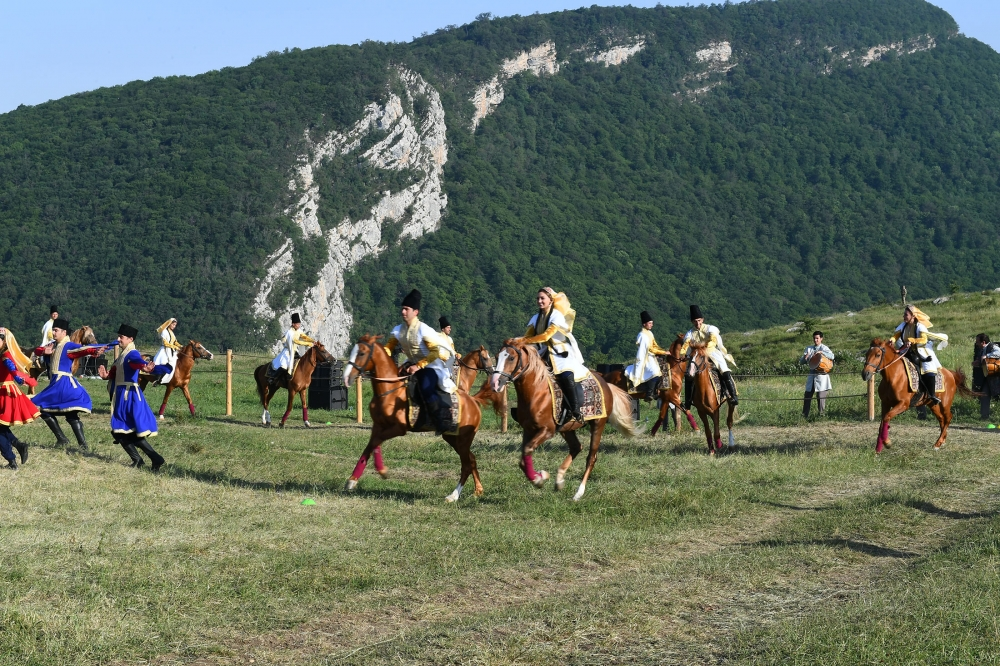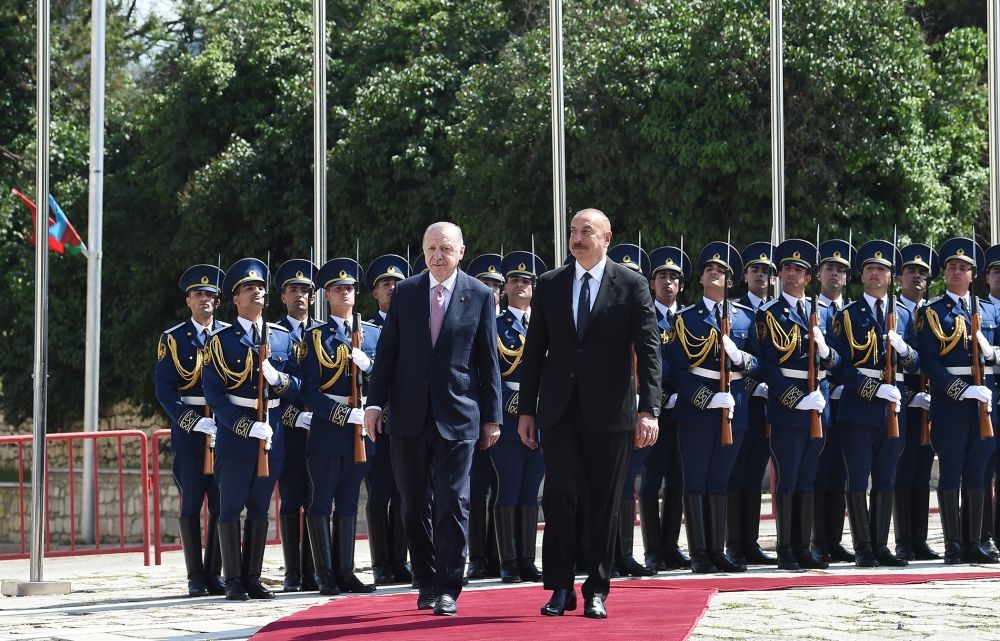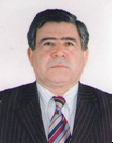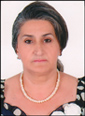Institute of Mathematics and Mechanics

## Karabakh news### “Musical heritage and Karabakh horses on Jidir Duzu plain” composition organized by Heydar Aliyev Foundation was presented in Shusha### Azerbaijan, Turkey signed Shusha Declaration on allied relations### Official welcome ceremony was held for Turkish President Recep Tayyip Erdogan in ShushaMisir Mardanov – Chief Director,
Corr.-member of ANAS,
Former Minister of Education of Azerbaijan Republic,
Doctor of Physical and Mathematical Sciences, Professor
Phone: (994 12) 5393924
Email: misir.mardanov@imm.azAdalet Yavuz oglu Akhundov  – Deputy Director
Deputy Director on science
Doctor of Sciences in Mathematics, Professor
Phone: (994 12) 5397579Tamilla Hasanova – Scientific secretary
Ph.D. in Physical and Mathematical Sciences, docent
Phone: (994 12) 5399192
Email: tamilla-togrul16@yahoo.com

Main area of activity of organization
In the field of mathematics: spectral analysis of operators and operator algebres; functional spaces and theory of functions; problems of harmonic analysis; differential equations and problem of mathematical physics; history of mathematical logic and mathematics.

Main scientific achievements made over the last five years:
Mathematical analysis.The boundedness theorems in the spaces of continuous and summable functions were proved for potential type operators and singular integrals depending on the generalized shift generated by Bessel differential operators and embedding theorems were established in Sobolev spaces and Sobolev weight spaces generated by Bessel operator.
Necessary basicity condition of power system of the functions of the form in the spaces was first found. Some analogy of the ” – Kadets” theorem in spaces at was found for perturbed system of exponents. A problem on finding analogies of the ” – Kadets” theorem on the basicity of a system of exponents for sines, cosines systems posed by Sedletskii in 1988 was completely solved. N.K.Bari’s classical theorem on Riesz basicity of close systems in Hilbert spaces that is transferred to Banach case, is revised.
In 1977 Yu. J. Kazmin pointed to impossibility of classical Stone- Weierstrass approximation theorem to some systems, where linear shell is not algebra. Generalization of the mentioned theorem was found for the complex case that is applied to Yu.J.Kazmin case.
Sufficient conditions and compactness and nucleus property creation of weight composition operators, and also for weight composition type integral operators, induced by holomorphic vector poles in uniform close subspaces of continuous functions on compacts are also given.

Differential equations.The direct and inverse scattering problems for a system of hyperbolic and ordinary differential equations were studied. A unique renewal of coefficients with respect to scattering operator for the considered system was proved. In some cases the scattering data were introduced.

A unique solvability of mixed problems and quality properties of their solutions in unbounded multivariate cylindric domains was researched for Sobolev type equations.

Algebra. The existence of invariant subspaces of semi – groups and Lie algebra of quasinilpotent compact operators was proved. The joint spectral radius formulae were obtained, new topological radicals were defined.

Approximation theory. A formula for calculation of the best approximation was found and extremal function was constructed. The jump problem was solved in a class of generalized analytic functions.

Fluid and gas mechanics. The possibility of estimation of parameters of unbalanced structures arising at fluid displacement in porous medium by means of physical parameters of such media is shown. The problem is theoretically solved on the basis of dependence of resistance factor on the frequency of applied pressure.
The possibility of regulation of development of unbalanced fractal structures by means of local pressure at the interface is proved, and oilgasfield technological method was worked out for the realization of the obtained effect.
Stability and oscillations analysis method of non-homogeneous elastico – plastic structural elements with regard for environment is worked out.

Mechanics of deformable solid. Analytic solution of nonlinear inverse boundary problem of axially symmetric large elastic deformations of circular orthographic membranes accepting preassigned form of a segment of rotation of a body under the action of fluid and normal loading is obtained.
The problem on eigen oscillation of a circular cylindric shell strengthened by a longitudinal rigidity ribs situation at the same distance from one another and filled by linear elastic medium, is solved. Under homogeneous boundary conditions on the ends the problem on stress -strain state of variable thickness transversally isotropic plate restricted by two conic and two spherical surfaces at its axially symmetric stretch – compression, is solved.
Equations of net motion in natural coordinates were derived.
Strong break front at self – model motion was found.
The problem on unstationary waves propagation in right prism was first considered and exact analytic solutions were found for concrete cases. Some facts discovered previously only experimentally for example, fact on propagation of main energy along the axis with rod velocity involving free lateral surfaces and etc. were affirmed theoretically.
Motion of circular inclusion containing elastically fixed mass with acoustic and elastic medium was studied.
Velocity potential, forces of medium reaction, elastic potentials and inclusions displacements were also determined.
Effective mathematical theory of cracking of corroding materials under mechanical stress was worked out.
The theorems admitting to represent the solutions of the problems of linear and a class of nonlinear elasticity theory by the solutions of the corresponding problem of elasticity theory were proved.
Mathematical theory of simulation of destruction of constructions made of visco – elasticoplastic material was suggested.

Statistics on COVID-19 in Azerbaijan
• Infected with the virus

479814

• Healed

449047

• Newly infected

1099

• Active patient

24334

• The state of death

6433

• Tested

4,814,503